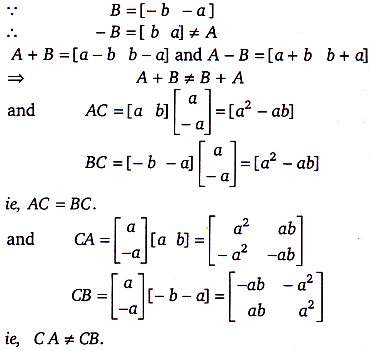Courses
Courses for Kids
Free study material
Free LIVE classes
More# If $A = \left[ {\begin{array}{*{20}{c}} a&b \end{array}} \right],\,B = \left[ {\begin{array}{*{20}{c}} { - b}&{ - a} \end{array}} \right]\,$ and $C = \left[ {\begin{array}{*{20}{c}} a \\ { - a} \end{array}} \right]$ then the correct statement isVerified